Win up to 100% scholarship on Aakash BYJU'S JEE/NEET courses with ABNAT Win up to 100% scholarship on Aakash BYJU'S JEE/NEET courses with ABNAT

# JEE Main 2022 June 28 – Shift 1 Physics Question Paper with Solutions

The JEE Main 2022 June 28 – Shift 1 Physics Question Paper with Solutions is available for reference and learning on this page. Our subject experts have examined and answered each of the questions from the Physics section. As a result, the answers have been prepared in a detailed manner. Candidates can also download the questions and solutions document in PDF format for offline use. JEE aspirants can use this study resource to prepare effectively for the JEE exam.

## JEE Main 2022 June 28th Physics Shift 1 Question Paper and Solutions

#### SECTION – A

Multiple Choice Questions: This section contains 20 multiple choice questions. Each question has 4 choices (1), (2), (3) and (4), out of which ONLY ONE is correct.

1. Given below are two statements : One is labelled as Assertion A and the other is labelled as Reason R.

Assertion A : Product of Pressure (P) and time (t) has the same dimension as that of coefficient of viscosity.

ReasonR : Coefficient of viscosity

$$\begin{array}{l}=\frac{\text{Force}}{\text{Velocity~gradient}}\end{array}$$

Choose the correct answer from the options given below.

(A) Both A and R true, and R is correct explanation of A.

(B) Both A and R are true but R is NOT the correct explanation of A.

(C) A is true but R is false.

(D) A is false but R is true.

Sol.

$$\begin{array}{l}\left [ \textup{Pressure} \right ] \left [ \textup{Time} \right ]=\left [\frac{\textup{Force}}{\textup{Area}} \right ]\left [ \frac{\textup{Distance}}{\textup{Velocity}} \right ]\end{array}$$
$$\begin{array}{l}\left [\textup{Coefficient~of~viscosity} \right ]=\left [\frac{\textup{Force}}{\textup{Area}}\right ]\left [\frac{\textup{Distance}}{\textup{Velocity}} \right ]\end{array}$$

Statement ‘A’ is true

But statement R is false are coefficient of viscosity

$$\begin{array}{l}=\dfrac{\text{Force}}{\text{Area }\times \text{Velocity gradient}}\end{array}$$

2. A particle of mass m is moving in a circular path of constant radius r such that its centripetal acceleration (a) is varying with time t as a = k2rt2, where k is a constant. The power delivered to the particle by the force acting on it is given as

(A) Zero

(B) mk2r2t2

(C) mk2r2t

(D) mk2rt

Sol.

$$\begin{array}{l}a_r=k^2rt^2=\frac{v^2}{r}\end{array}$$
$$\begin{array}{l}\Rightarrow v^2=k^2r^2t^2~~\textup{or}~~v=krt \end{array}$$

and

$$\begin{array}{l}\frac{d\left|v \right|}{dt}=kr \end{array}$$

at = kr

$$\begin{array}{l}\Rightarrow \left|\vec{F}\cdot\vec{v} \right|=\left ( mkr \right )\left ( krt \right )\end{array}$$

= mk2r2t = power delivered

3. Motion of a particle in xy plane is described by a set of following

$$\begin{array}{l}x=4\sin\left ( \frac{\pi}{2}-\omega t\right )~\text{m}\end{array}$$
and y = 4sin(ωt) m. The path of the particle will be

(A) Circular

(B) Helical

(C) Parabolic

(D) Elliptical

Sol.

$$\begin{array}{l}x=4\sin\left ( \frac{\pi}{2}-\omega t \right )\end{array}$$

= 4cos(ωt)

y = 4 sin(ωt)

x2 + y2 = 42

⇒ The particle is moving in a circular motion with radius of 4 m.

4. Match List-I with List-II.

 List-I List-II A. Moment of inertia of solid sphere of radius R about any tangent I. $$\begin{array}{l}\frac{5}{3}MR^2 \end{array}$$ B. Moment of inertia of hollow sphere of radius (R) about any tangent. II. $$\begin{array}{l}\frac{7}{5}MR^2 \end{array}$$ C. Moment of inertia of circular ring of radius (R) about its diameter. III. $$\begin{array}{l}\frac{1}{4}MR^2 \end{array}$$ D. Moment of inertia of circular disc of radius (R) about any diameter. IV. $$\begin{array}{l}\frac{1}{2}MR^2 \end{array}$$

Choose the correct answer from the options given below.

(A) A-II, B-I, C-IV, D-III

(B) A-I, B-II, C-IV, D-III

(C) A-II, B-I, C-III, D-IV

(D) A-I, B-II, C-III, D-IV

Sol. (A) Moment of inertia of solid sphere of radius R about a tangent

$$\begin{array}{l}=\frac{2}{5}MR^2+MR^2=\frac{7}{5}MR^2 \end{array}$$

⇒ A – (II)

(B) Moment of inertia of hollow sphere of radius R about a tangent

$$\begin{array}{l}=\frac{2}{3}MR^2+MR^2=\frac{5}{3}MR^2 \end{array}$$

⇒ B – (I)

(C) Moment of inertia of circular ring of radius (R) about its diameter

$$\begin{array}{l}=\frac{\left ( MR^2 \right )}{2} \end{array}$$

⇒ C – (IV)

(D) Moment of inertia of circular ring of radius (R) about any diameter

$$\begin{array}{l}=\frac{MR^2/2}{2}=\frac{MR^2}{4} \end{array}$$

⇒ D – (III)

5. Two planets A and B of equal mass are having their period of revolutions TAandTBsuch that TA= 2TB. These planets are revolving in the circular orbits of radii rAandrBrespectively. Which out of the following would be the correct relationship of their orbits?

$$\begin{array}{l}(A)\ 2r_A^2=r_B^3\end{array}$$
$$\begin{array}{l}(B)\ r_A^3=2r_B^3\end{array}$$
$$\begin{array}{l}(C)\ r_A^3=4r_B^3\end{array}$$
$$\begin{array}{l}(D)\ T_A^2-T_B^2=\frac{\pi^2}{GM}\left ( r_B^3-4r_A^3 \right ) \end{array}$$

Sol. TA = 2TB

Now

$$\begin{array}{l}T_A^2 \propto r_A^3\end{array}$$
$$\begin{array}{l}\Rightarrow \left ( \frac{r_A}{r_B} \right )^3 = \left ( \frac{T_A}{T_B} \right )^2\end{array}$$
$$\begin{array}{l}\Rightarrow r_A^3=4r_B^3 \end{array}$$

6. A water drop of diameter 2 cm is broken into 64 equal droplets. The surface tension of water is 0.075 N/m. In this process the gain in surface energy will be

(A) 2.8 × 10–4 J

(B) 1.5 × 10–3 J

(C) 1.9 × 10–4 J

(D) 9.4 × 10–5 J

Sol.

$$\begin{array}{l}r’=\frac{r}{4}\end{array}$$

⇒ ΔE = TS)

= T × 4π(nr2r2), n = 64

= T × 4π × (4 – 1)r2

⇒ ΔE = 0.075 × 4 × 3.142 (3) × 10–4 J

= 2.8 × 10–4 J

7. Given below are two statements

Statement-I: When μ amount of an ideal gas undergoes adiabatic change from state (P1, V1, T1) to state (P2, V2, T2), then work done is

$$\begin{array}{l}W=\frac{\mu R\left ( T_2-T_1 \right )}{1-\gamma}\end{array}$$
, where
$$\begin{array}{l}\gamma=\frac{C_p}{C_v}~\textup{and}~R =\text{universal gas constant}.\end{array}$$

Statement-II: In the above case, when work is done on the gas, the temperature of the gas would rise.

Choose the correct answer from the options given below.

(A) Both statement-I and statement-II are true

(B) Both statement-I and statement-II are false

(C) Statement-I is true but statement-II is false

(D) Statement-I is false but statement-II is true

Sol.

$$\begin{array}{l}W=\frac{\mu R\left ( T_2-T_1 \right )}{1-r} \end{array}$$
for a polytropic process for adiabatic process r = γ

⇒ Statement I is true

ΔU = –ΔW

⇒ If work is done on the gas

⇒ ΔW is negative

⇒ ΔU is positive or temperature increases

⇒ Statement II is true

8. Given below are two statements

Statement-I: A point charge is brought in an electric field. The value of electric field at a point near to the charge may increase if the charge is positive.

Statement-II: An electric dipole is placed in a non-uniform electric field. The net electric force on the dipole will not be zero.

Choose the correct answer from the options given below.

(A) Both statement-I and statement-II are true

(B) Both statement-I and statement-II are false

(C) Statement-I is true but statement-II is false

(D) Statement-I is false but statement-II is true

Sol. As one moves closer to a positive charge (isolated) the density of electric field line increases and so does the electric field intensity

⇒ Statement I is true

As opposite poles of an electric dipole would experience equal and opposite forces so net force on a dipole in a uniform electric field will be zero

⇒ Statement II is true

9. The three charges q/2, q and q/2 are placed at the corners A, B and C of a square of side ‘a’ as shown in figure. The magnitude of electric field (E) at the corner D of the square is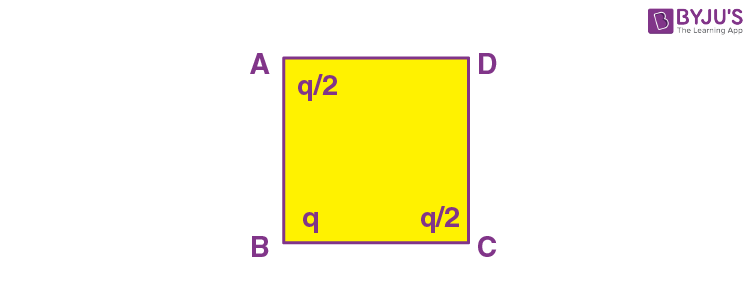(A)

$$\begin{array}{l}\frac{q}{4\pi\epsilon_0a^2}\left ( \frac{1}{\sqrt{2}}+\frac{1}{2} \right )\end{array}$$

(B)

$$\begin{array}{l}\frac{q}{4\pi\epsilon_0~a^2}\left ( 1+\frac{1}{\sqrt{2}} \right )\end{array}$$

(C)

$$\begin{array}{l}\frac{q}{4\pi\epsilon_0a^2}\left ( 1-\frac{1}{\sqrt{2}} \right )\end{array}$$

(D)

$$\begin{array}{l}\frac{q}{4\pi\epsilon_0a^2}\left ( \frac{1}{\sqrt{2}}-\frac{1}{2} \right )\end{array}$$

Sol.

$$\begin{array}{l}\left|E_0 \right|=\frac{kq/2}{a^2}\sqrt{2}+\frac{kq}{\left ( a\sqrt{2} \right )^2}\end{array}$$
$$\begin{array}{l}=\frac{kq}{\sqrt{2}a^2}+\frac{kq}{2a^2}\end{array}$$
$$\begin{array}{l}=\frac{kq}{a^2}\left ( \frac{1}{\sqrt{2}}+\frac{1}{2} \right ),k=\frac{1}{4\pi\epsilon_0} \end{array}$$

⇒ Option A is correct

10. An infinitely long hollow conducting cylinder with radius R carries a uniform current along its surface.

Choose the correct representation of magnetic field (B) as a function of radial distance (r) from the axis of cylinder.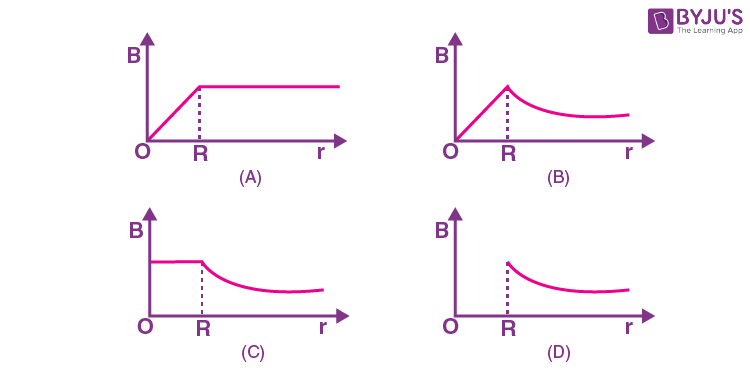Sol. Inside a hollow cylindrical conductor with uniform current distribution net magnetic field is zero in hollow space.

But outside the cylindrical conductor

$$\begin{array}{l}B\propto\frac{1}{r}\end{array}$$

⇒ Graph in option D would be a correct one

11. A radar sends an electromagnetic signal of electric field (E0) = 2.25 V/m and magnetic field (B0) = 1.5 × 10–8 T which strikes a target on line of sight at a distance of 3 km in a medium. After that, a part of signal (echo) reflects back towards the radar with same velocity and by same path. If the signal was transmitted at time t = 0 from radar, then after how much time echo will reach to the radar?

(A) 2.0 × 10–5 s

(B) 4.0 × 10–5 s

(C) 1.0 × 10–5 s

(D) 8.0 × 10–5 s

Sol. E0 = 2.25 V/m

B0 = 1.5 × 10–8T

$$\begin{array}{l}\Rightarrow \frac{E_0}{B_0}=1.5\times10^8~\text{m/s}\end{array}$$

⇒ Refractive index = 2

Distance to be travelled = 6 km

Time taken

$$\begin{array}{l}=\frac{6\times10^3}{1.5\times10^8}=4\times10^{-5}~\text{s}\end{array}$$

⇒ Option (B) is correct

12. The refracting angle of a prism is A and refractive index of the material of the prism is cot (A/2). Then the angle of minimum deviation will be :

(A) 180 – 2A

(B) 90 – A

(C) 180 + 2A

(D) 180 – 3A

Sol.

$$\begin{array}{l}\mu=\frac{\sin\left ( \frac{\delta_m+A}{2} \right )}{\sin\left ( A/2 \right )}=\cot A/2 \end{array}$$
$$\begin{array}{l}\Rightarrow \cos A/2=\sin\left ( \frac{\delta_m+A}{2} \right )\end{array}$$
$$\begin{array}{l}\Rightarrow \frac{\pi}{2}-\frac{A}{2}=\frac{\delta_m+A}{2}\end{array}$$

⇒ π – 2A = δm

Option (A) is correct

13. The aperture of the objective is 24.4 cm. The resolving power of this telescope, if a light of wavelength 2440 Å is used to see the object will be:

(A) 8.1 × 106

(B) 10.0 × 107

(C) 8.2 × 105

(D) 1.0 × 10–8

Sol.

$$\begin{array}{l}R.P.= \frac{1}{1.22\lambda/a}\end{array}$$
$$\begin{array}{l}=\frac{24.4\times 10^{-2}}{1.22\times 2440\times 10^{-10}} \end{array}$$

= 8.2 × 105

Option (C) is correct

14. The de Broglie wavelengths for an electron and a photon are λe and λp, respectively. For the same kinetic energy of electron and photon, which of the following presents the correct relation between the de Broglie wavelengths of two?

$$\begin{array}{l}(A)\ \lambda_p\propto\lambda_e^2\end{array}$$
$$\begin{array}{l}(B)\ \lambda_p\propto\lambda_e \end{array}$$
$$\begin{array}{l}(C)\ \lambda_p\propto\sqrt{\lambda_e} \end{array}$$
$$\begin{array}{l}(D)\ \lambda_p\propto\sqrt{\frac{1}{\lambda_e}} \end{array}$$

Sol.

$$\begin{array}{l}\lambda_p=\frac{h}{p}=\frac{hc}{E}…(i) \end{array}$$
$$\begin{array}{l}\lambda_e=\frac{h}{\sqrt{2mE}}…(ii)\end{array}$$

From (i) and (ii)

$$\begin{array}{l}\lambda_p\propto\lambda_e^2\end{array}$$

⇒ Option A is correct

15. The Q-value of a nuclear reaction and kinetic energy of the projectile particle, Kp are related as :

(A) Q = Kp

(B) (Kp + Q) < 0

(C) Q < Kp

(D) (Kp + Q) > 0

Sol. Kp> 0

If Q is released ⇒Q> 0

Kp + Q> 0

If Q is absorbed ⇒Q< 0

Even then particle has to be given kinetic energy greater than magnitude of Q to maintain momentum conservation.

K + Q> 0

⇒ Option D is correct

16. In the following circuit, the correct relation between output (Y) and inputs A and B will be: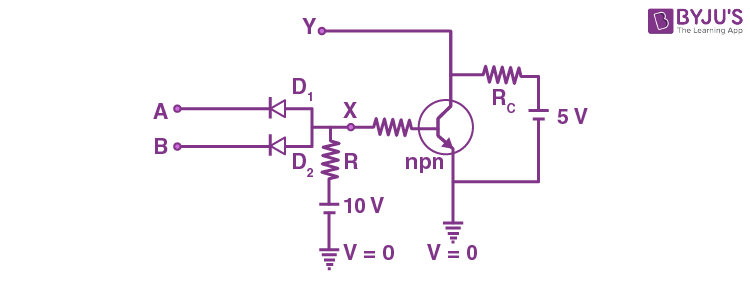(A) Y = AB

(B) Y = A + B

$$\begin{array}{l}(C)\ Y=\overline{AB} \end{array}$$
$$\begin{array}{l}(D)\ Y=\overline{A+B} \end{array}$$

Sol. The shown circuit is a combination of AND gate and a NOT gate.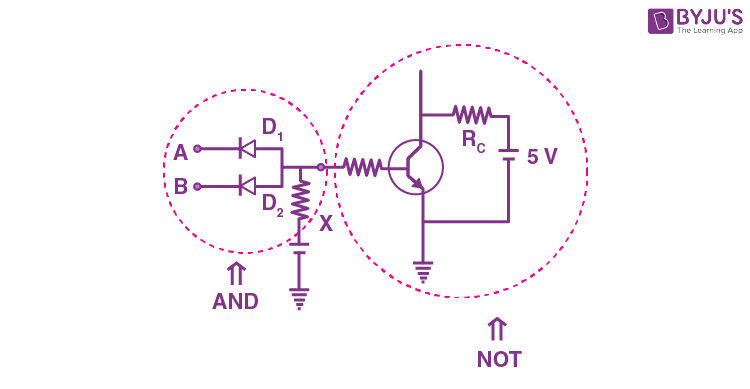$$\begin{array}{l}\Rightarrow Y = \overline{AB} \end{array}$$

Option (C) is a correct option.

17. For using a multimeter to identify diode from electrical components, choose the correct statement out of the following about the diode:

(A) It is two terminal device which conducts current in both directions.

(B) It is two terminal device which conducts current in one direction only

(C) It does not conduct current gives an initial deflection which decays to zero.

(D) It is three terminal device which conducts current in one direction only between central terminal and either of the remaining two terminals.

Sol. A diode is a two terminal device which conducts current in forward bias only

Option (B) is correct.

18. Given below are two statements: One is labelled as Assertion A and the other is labelled as Reason R.

Assertion A :n-p-n transistor permits more current than a p-n-p transistor.

Reason R: Electrons have greater mobility as a charge carrier.

Choose the correct answer from the options given below:

(A) Both A and Rare true, and R is correct explanation of A.

(B) Both A and R are true but R is NOT the correct explanation of A.

(C) A is true but R is false.

(D) A is false but R is true.

Sol. (A) is true as n-p-n transistor permits more current than p-n-p transistor as electrons which are majority charge carriers in n-p-n have higher mobility than holes which are majority carriers in

p-n-p transistor

Statement R is correct explanation of statement A

19. Match List-I with List-II.

 List-I List-II (A) Television signal I. 03 KHz (B) Radio signal II. 20 KHz (C) High Quality Music III. 02 MHz (D) Human speech IV. 06 MHz

Choose the correct answer from the options given below:

(A) A-I, B-II, C-III, D-IV

(B) A-IV, B-III, C-I, D-II

(C) A-IV, B-III, C-II, D-I

(D) A-I, B-II, C-IV, D-III

Sol. Television signal ⇒ 6 MHz

High Quality music ⇒ 20 kHz

Human speech ⇒ 3 kHz

Option (C) is correct.

20. The velocity of sound in a gas, in which two wavelengths, 4.08 m and 4.16 m produce 40 beats in 12 s, will be:

(A) 282.8 ms–1

(B) 175.5 ms–1

(C) 353.6 ms–1

(D) 707.2 ms–1

Sol.

$$\begin{array}{l}\frac{v}{4.08}-\frac{v}{4.16}=\frac{40}{12}\end{array}$$
$$\begin{array}{l}v=\frac{40}{12}\times\frac{4.08\times4.16}{0.08}\end{array}$$

= 707.2 m/s

Option (D) is correct.

#### SECTION – B

Numerical Value Type Questions: This section contains 10 questions. In Section B, attempt any five questions out of 10. The answer to each question is a NUMERICAL VALUE. For each question, enter the correct numerical value (in decimal notation, truncated/rounded-off to the second decimal place; e.g. 06.25, 07.00, –00.33, –00.30, 30.27, –27.30) using the mouse and the on-screen virtual numeric keypad in the place designated to enter the answer.

1. A pendulum is suspended by a string of length 250 cm. The mass of the bob of the pendulum is 200 g. The bob is pulled aside until the string is at 60° with vertical, as shown in the figure. After releasing the bob, the maximum velocity attained by the bob will be ___ ms–1. (if g = 10 m/s2)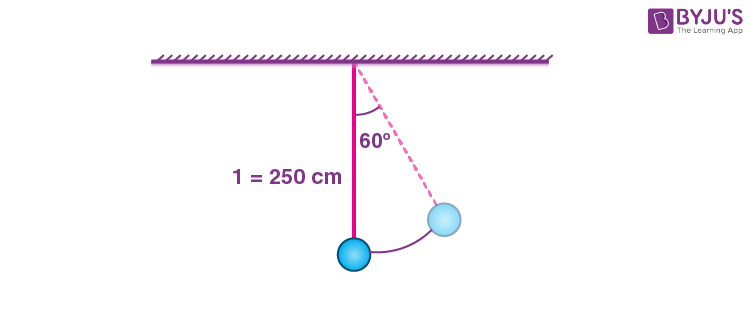Sol.

$$\begin{array}{l}\frac{1}{2}mv^2=mgl\left ( 1-\cos\theta \right ) \end{array}$$
$$\begin{array}{l}\Rightarrow v=\sqrt{2gl\left ( 1-\cos\theta \right )} \end{array}$$
$$\begin{array}{l}=\sqrt{2\times10\times2.5\times\frac{1}{2}} \end{array}$$

= 5 m/s

2. A meter bridge setup is shown in the figure. It is used to determine an unknown resistance R using a given resistor of 15 Ω. The galvanometer (G) shows null deflection when tapping key is at 43 cm mark from end A. If the end correction for end A is 2 cm, then the determined value of R will be ___ Ω.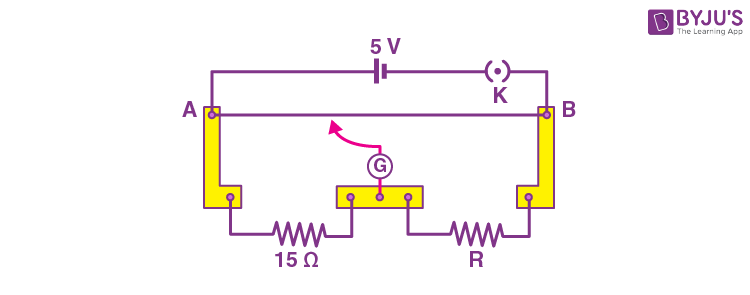Sol.

$$\begin{array}{l}\frac{43+2}{15}=\frac{57}{R} \end{array}$$
$$\begin{array}{l}R=\frac{57\times15}{45}=19\Omega \end{array}$$

3. Current measured by the ammeter Ⓐ in the reported circuit when no current flows through 10 Ω resistance, will be ___ A.Sol. For I10 = 0

$$\begin{array}{l}\frac{R}{3}=\frac{4}{6} \end{array}$$

R = 2 Ω

$$\begin{array}{l}\Rightarrow I_A=\frac{36\times\left ( 6+9 \right )}{6\times9}\end{array}$$
$$\begin{array}{l}=\frac{36\times15}{6\times9}=10~\text{A}\end{array}$$

4. An AC source is connected to an inductance of 100 mH, a capacitance of 100 μF and a resistance of 120 Ω as shown in the figure. The time in which the resistance having a thermal capacity 2 J/°C will get heated by 16°C is ___ s.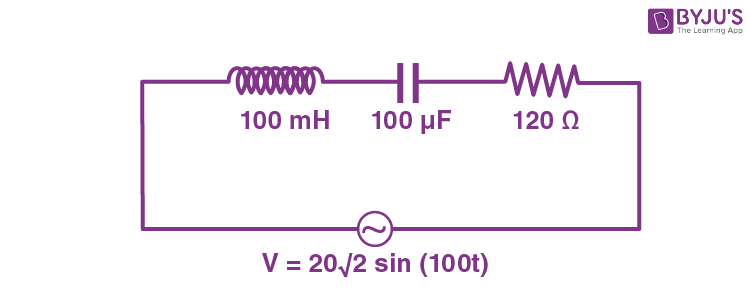Sol. L = 100 × 10–3H

C = 100 × 10–6 F

R = 120 Ω

ωL = 10 Ω

$$\begin{array}{l}\frac{1}{\omega C}=\frac{1}{10^4\times10^{-6}}=100\Omega\end{array}$$

XCXL = 90 Ω

$$\begin{array}{l}\Rightarrow Z=\sqrt{90^2+120^2}=150~\Omega\end{array}$$
$$\begin{array}{l}\Rightarrow I_{rms}=\frac{20}{150}=\frac{2}{15}~\text{A}\end{array}$$

For heat resistance by 16°C heat required = 32 J

$$\begin{array}{l}\Rightarrow \left ( \frac{2}{15} \right )^2\times\left ( 120 \right )\times t=32 \end{array}$$
$$\begin{array}{l}t=\frac{32\times15\times15}{4\times120} \end{array}$$

= 15

5. The position vector of 1 kg object is

$$\begin{array}{l}\vec{r} =\left ( 3\hat{i}-\hat{j}\right )~\text{m}\end{array}$$
and its velocity
$$\begin{array}{l}\vec{v}=\left ( 3\hat{j}+\hat{k}\right )\text{ms}^{-1}.\end{array}$$
The magnitude of its angular momentum is √x Nm where x is

Sol.

$$\begin{array}{l}\left|\vec{i} \right| =\left|\vec{r}\times\left ( m\vec{v} \right ) \right|\end{array}$$
$$\begin{array}{l}=\left|\left ( 3\hat{i}-\hat{j} \right )\times\left ( 3\hat{j}+\hat{k} \right ) \right|\end{array}$$
$$\begin{array}{l}=\left|-\hat{i}-3\hat{j}+9\hat{k} \right| \end{array}$$
$$\begin{array}{l}=\sqrt{91} \end{array}$$

6. A man of 60 kg is running on the road and suddenly jumps into a stationary trolly car of mass 120 kg. Then, the trolly car starts moving with velocity 2ms–1. The velocity of the running man was _____ ms–1, when he jumps into the car.

Sol.

$$\begin{array}{l}v_m=\frac{\left ( 120+60 \right )v_T}{60} \end{array}$$
$$\begin{array}{l}=\frac{180\times2}{60} \end{array}$$
= 6 m/s

7. A hanging mass M is connected to a four times bigger mass by using a string-pulley arrangement, as shown in the figure. The bigger mass is placed on ahorizontal ice-slab and being pulled by 2Mg force. In this situation, tension in the string is x/5 Mg for x= __________. Neglect mass of the string and friction of the block (bigger mass) with ice slab.

(Given g= acceleration due to gravity)Sol.

$$\begin{array}{l}a=\frac{Mg}{4M+M}=\frac{g}{5} \end{array}$$
(in upward direction)

$$\begin{array}{l}T=M\left ( g+\frac{g}{5} \right )=\frac{6Mg}{5} \end{array}$$

x = 6

8. The total internal energy of two mole monoatomic ideal gas at temperatureT=300 K will be _____J. (Given R = 8.31 J/mol.K)

Sol.

$$\begin{array}{l}U=2\left ( \frac{3}{2}R \right )300 \end{array}$$

= 3 × 8.31 × 300

= 7479 J

9. A singly ionized magnesium atom (A=24) ion is accelerated to kinetic energy 5keV and is projected perpendicularly into a magnetic field B of the magnitude 0.5 T. The radius of path formed will be ________ cm.

Sol.

$$\begin{array}{l}R=\frac{mv}{qB}\end{array}$$
$$\begin{array}{l}R=\frac{\sqrt{2mKE}}{qB}\end{array}$$
$$\begin{array}{l}=\frac{\sqrt{2\times24\times1.67\times10^{-27}\times5\times1.6\times10^{-16}}}{1.6\times10^{-19}\times0.5} \end{array}$$

=10.009 cm = 10 cm

10. A telegraph line of length 100 km has a capacity of 0.01μF/km, and it carries an alternating current at 0.5 kilo cycle per second. If the minimum impedance is required, then the value of the inductance that needs to be introduced in series is_______ mH.

(if π = √10)

Sol. Total capacitance = 0.01 × 100 = 1 μF

ω = 500 × 2π = 1000π rad/s

$$\begin{array}{l}\omega L=\frac{1}{\omega C} \end{array}$$
$$\begin{array}{l}\Rightarrow L=\frac{1}{\omega^2C}=\frac{1}{10^6\pi^2\times10^{-6}}=\frac{1}{10}H= 100~\text{mH}\end{array}$$

### Download PDF of JEE Main 2022 June 28 Shift 1 Physics Paper & Solutions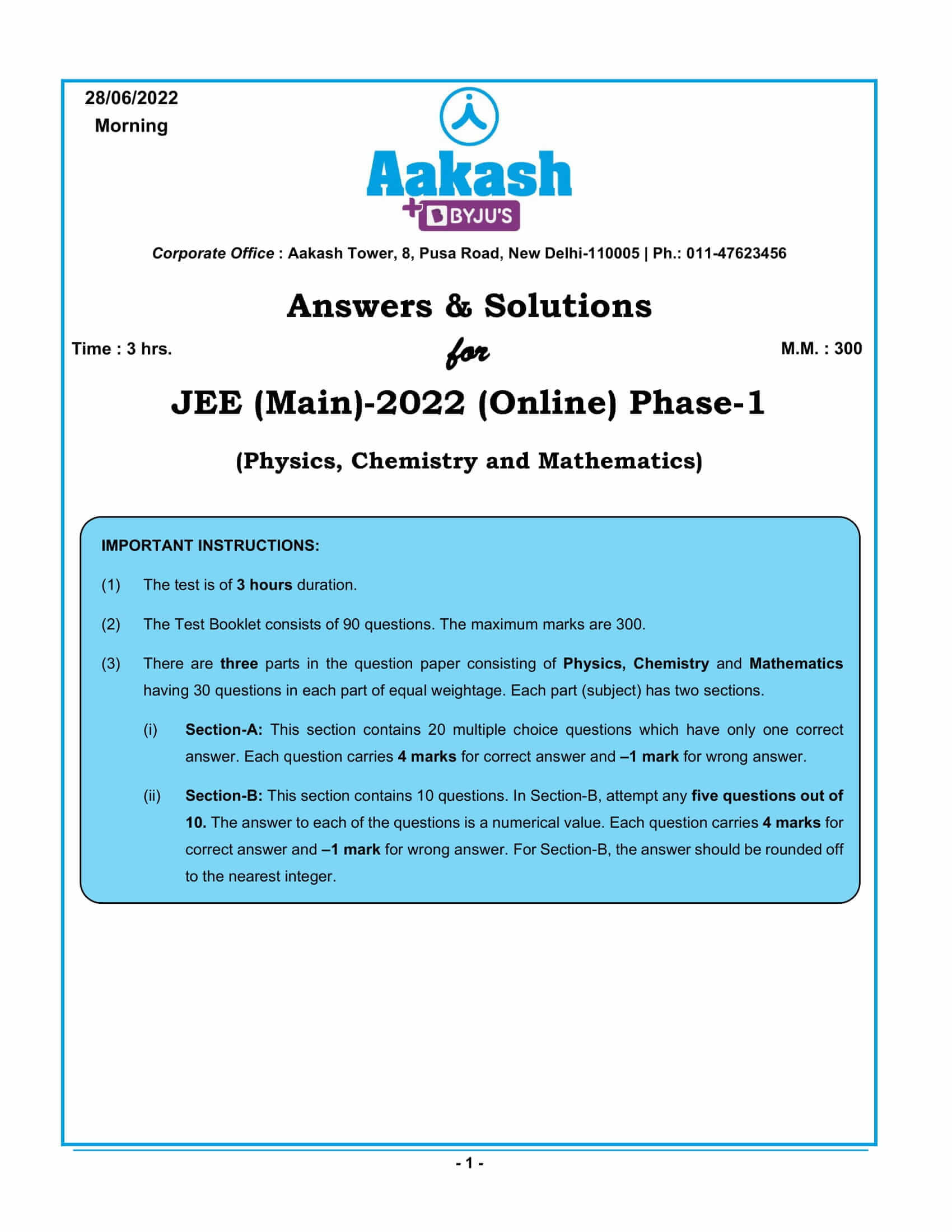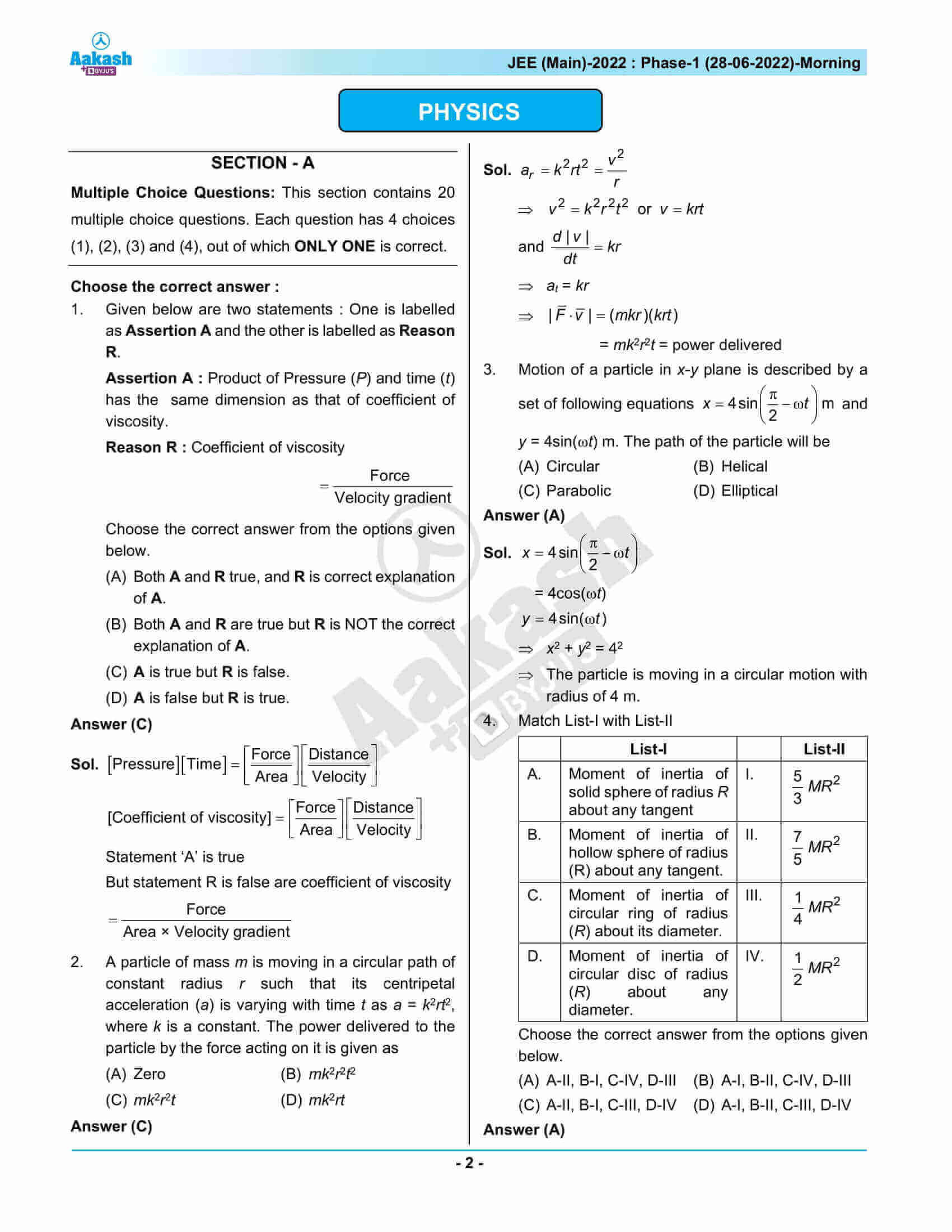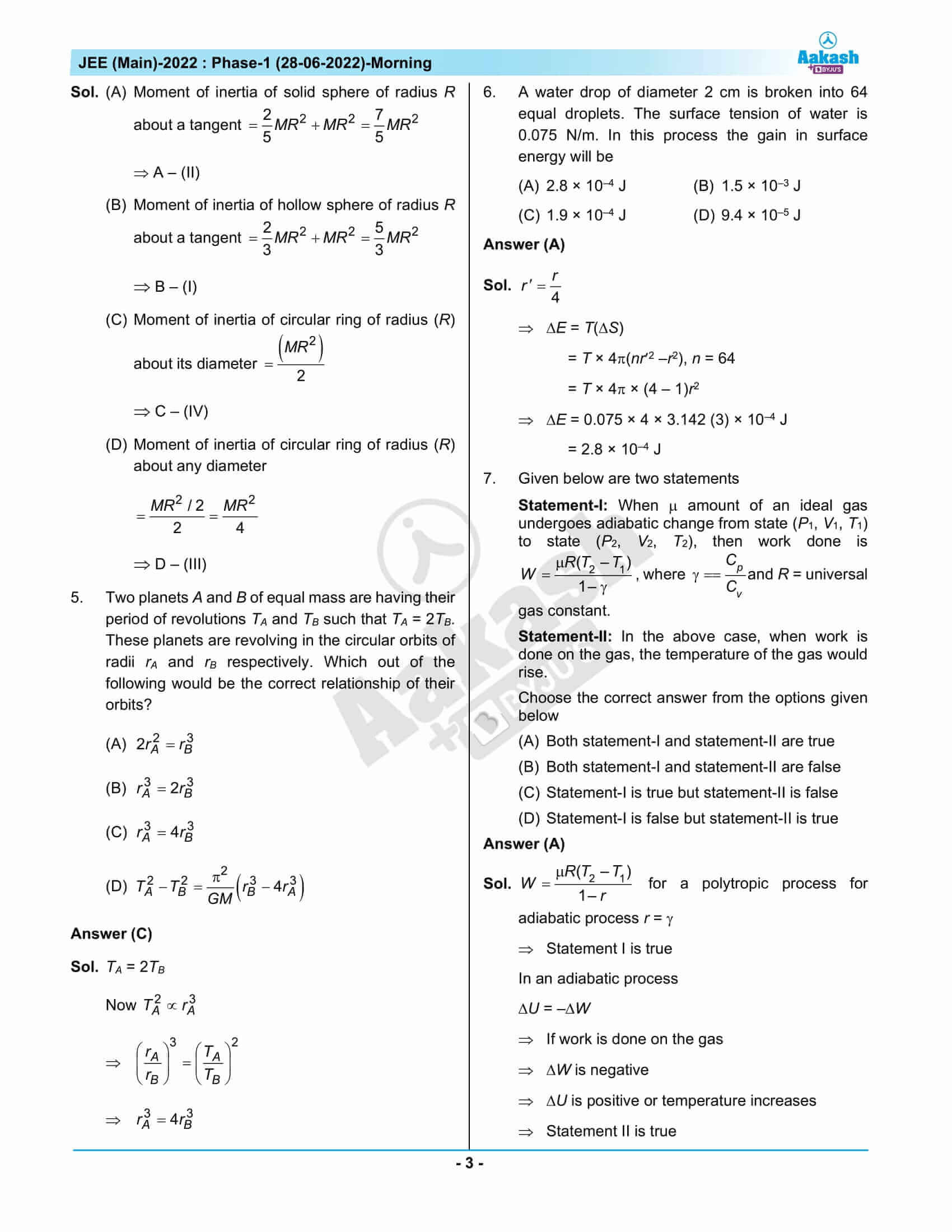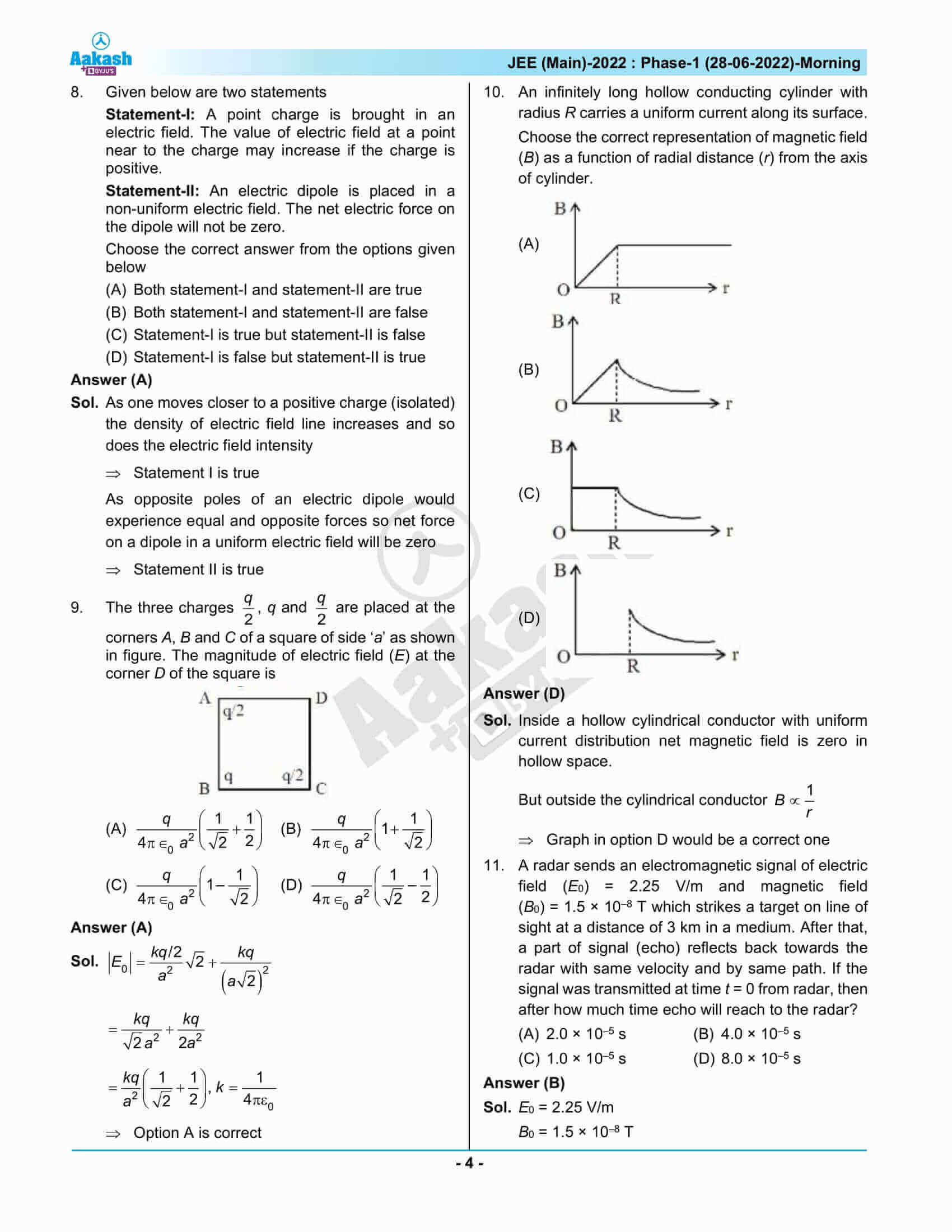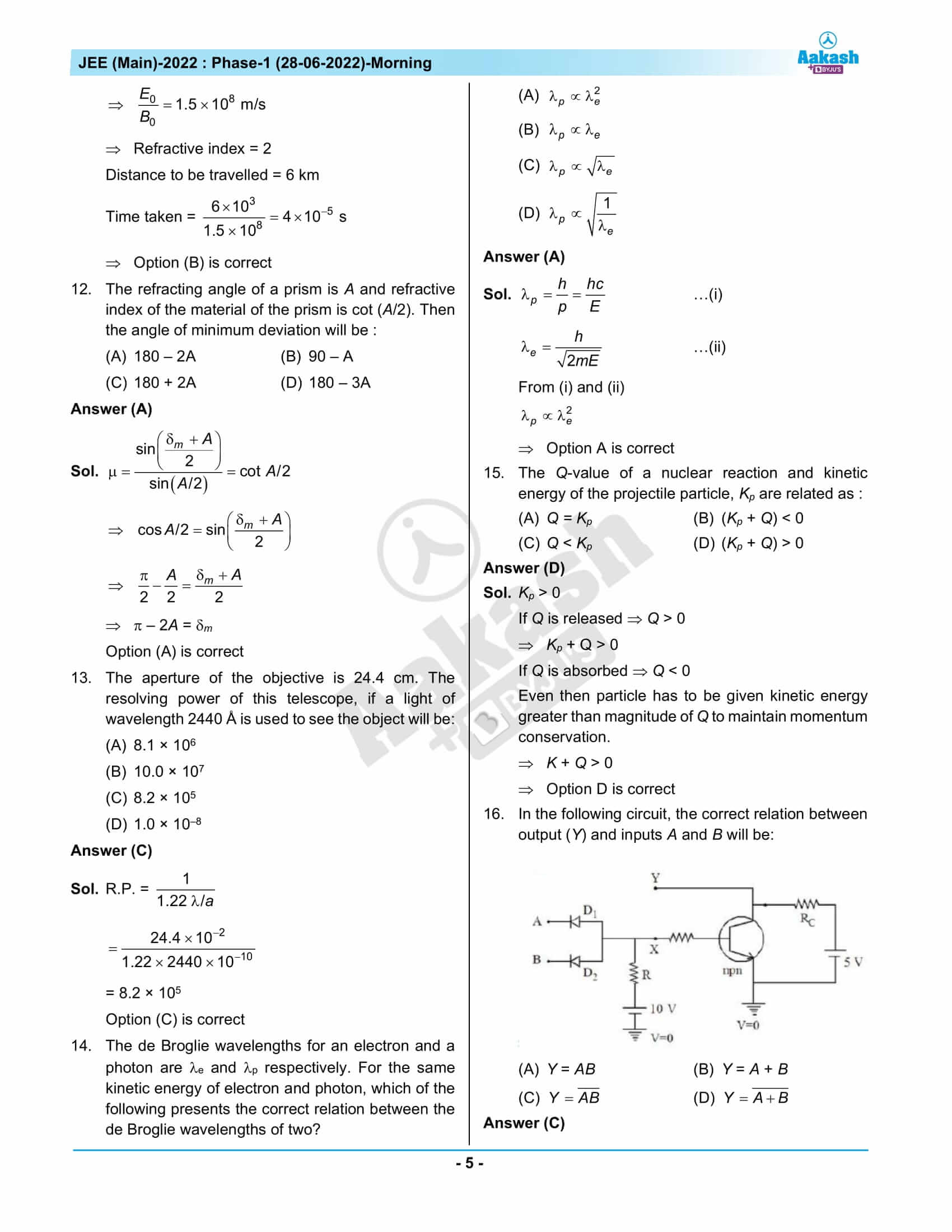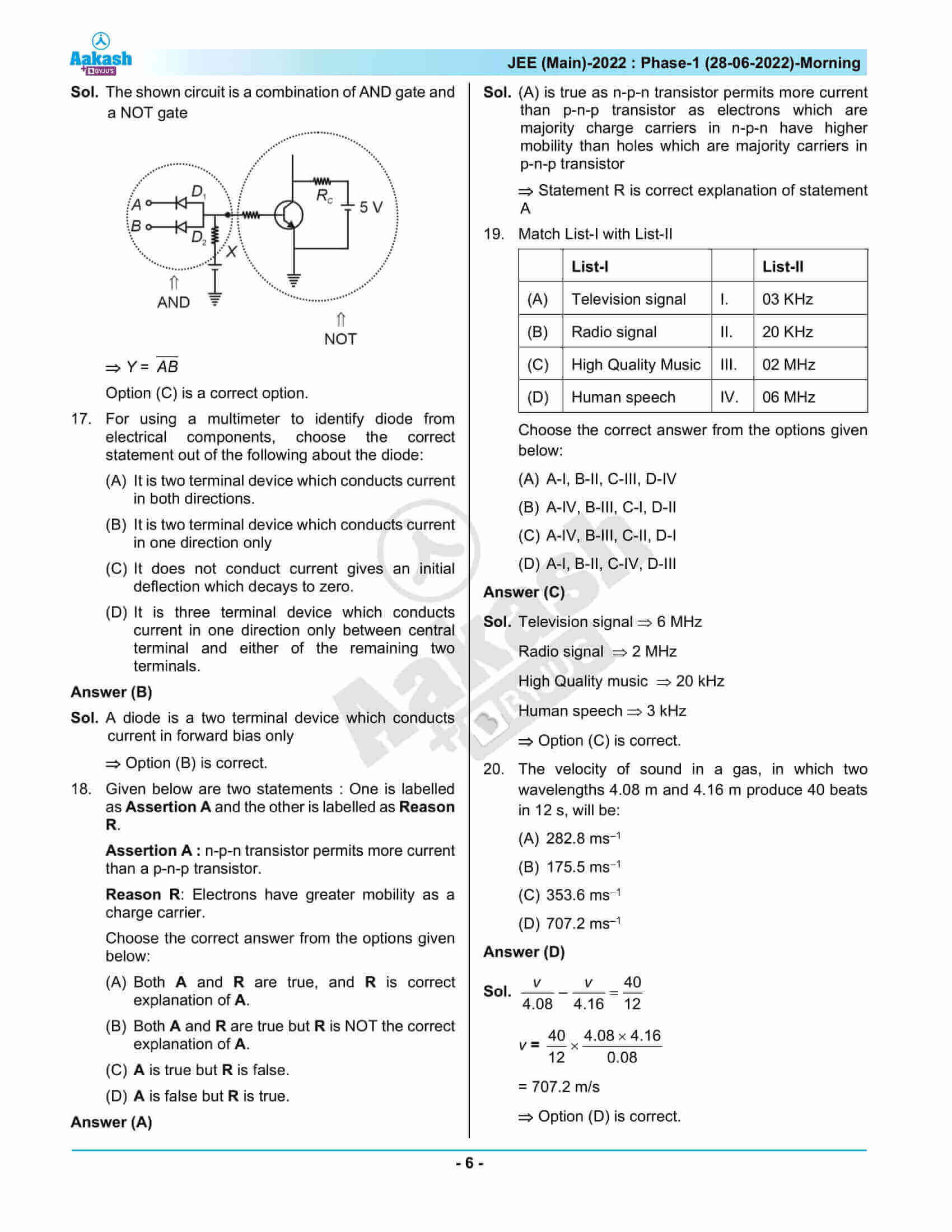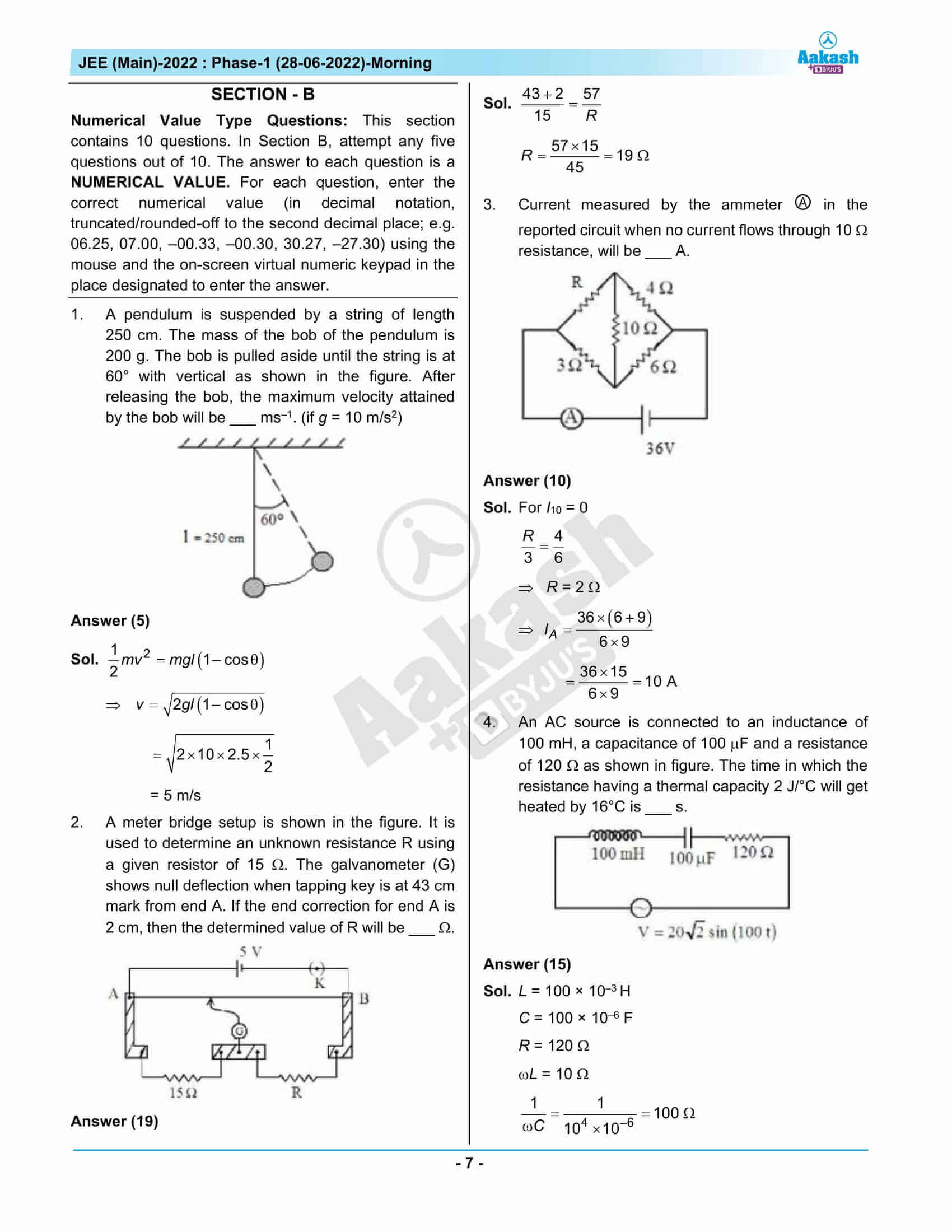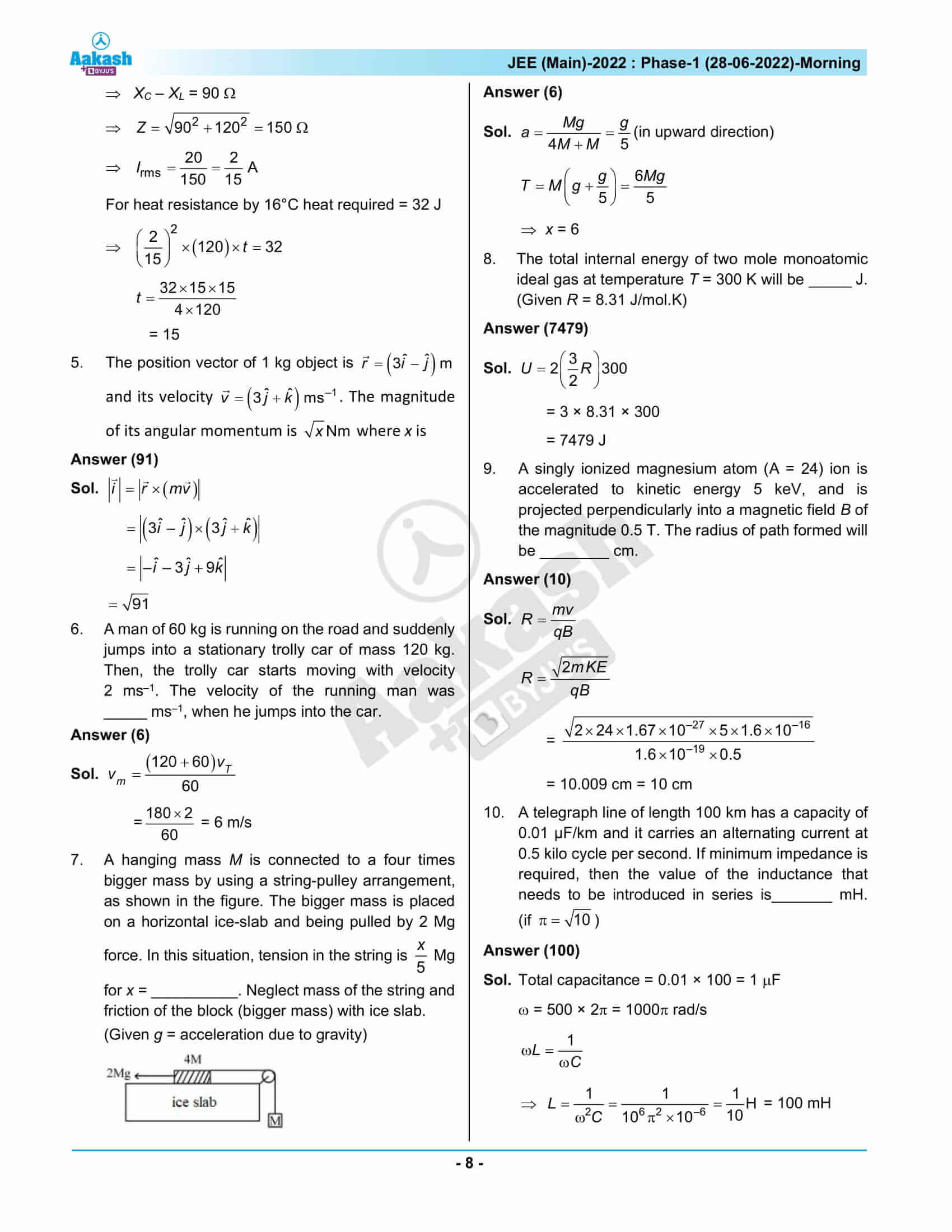## JEE Main 2022 Question Paper & Solutions – June 28th Shift 1

### JEE Main 2022 June 28 Shift 1 Question Paper – Physics Solutions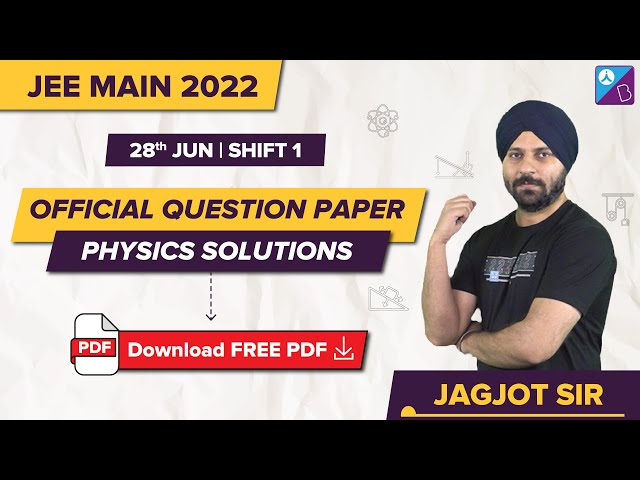### JEE Main 2022 June 28 Shift 1 Question Paper – Chemistry Solutions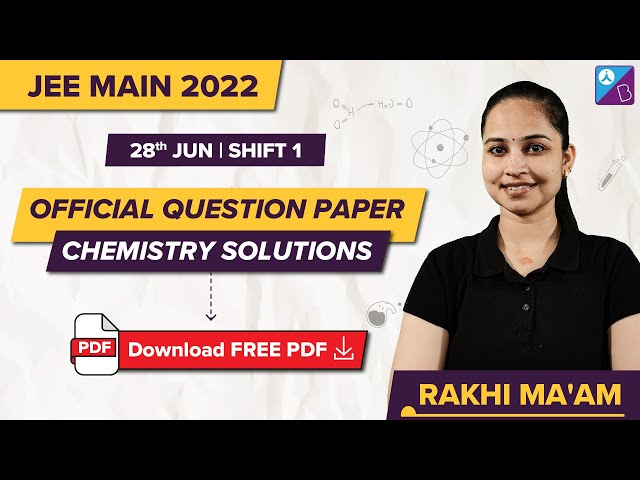### JEE Main 2022 June 28 Shift 1 Question Paper – Maths Solutions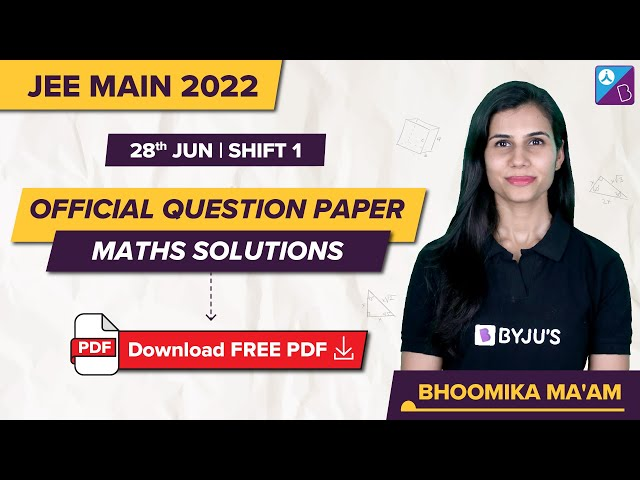## Frequently Asked Questions – FAQs

Q1

### What was the percentage of questions asked from Class 11 and Class 12 in the Physics section of JEE Main 2022, June 28 morning shift question paper?

Out of the 27 memory-based Physics questions, 13 were from the Class 11 curriculum and 14 from the Class 12 curriculum. Therefore, 43% of Physics questions were from Class 11 and 47% from Class 12.

Q2

### What was the nature of the questions asked in the Physics section of JEE Main 2022 June 28 shift 1 question paper?

Compared to Maths and Chemistry, Physics was easy. There were 18 easy questions in Physics and 9 medium-type questions.

Q3

### What were the topics included from the Class 12 Physics syllabus in JEE Main 2022 June 28 shift 1 question paper?

The topics included from the Class 12 Physics syllabus in JEE Main 2022 June 28 shift 1 question paper are:
Wheatstone Bridge
Resolving Power of Optical Instruments
Superposition
Junction Transistor
Electrostatic Potential Energy
Prism
Photoelectric Effect
Wave Nature of Matter
Magnetic Field Due To Various Current Carrying Conductors
Semiconductor Diode
Combination of Resistors – Series and Parallel
Refraction
Meter Bridge

Q4

### What were the Physics Class 11 chapters from which questions were included in JEE Main 2022, June 28 shift 1 question paper?

The Class 11 Physics chapters from which questions were asked in JEE Main 2022, June 28 shift 1 question paper are:
Semiconductor Electronics
Mechanical Properties of Fluids
Gravitation
Laws of Motion
System of Particles and Rotational Motion
Motion In A Plane
Thermodynamics
Work, Energy and Power
Kinetic Theory
Laws of Motion
Waves

Q5

### What was the difficulty level of Physics questions asked in JEE Main 2022, June 28 shift 1 question paper?

The difficulty level of the Physics question paper is rated as 1.33 on a scale of 1 to 3.